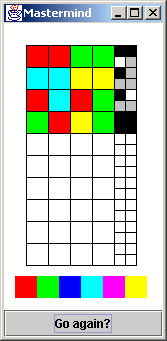# Mastermind

In Mastermind, player one selects a sequence of four colored pegs, and player two makes guesses until she has identified the sequence. There are six colors, and each color may be used more than once. As guesses are made, the feedback provided is: a black peg for each correct color in the correct position, and a white peg for each correct color in the wrong position.

A "command line" user interface for Mastermind is almost as enjoyable as the graphical UI.   And – implementing a "command line" UI is very straight-forward. The user is prompted for 4 integers, and then receives feedback in the form of 2 integers: the number of black pegs, and the number of white pegs.

This "game" is an excellent project for teaching programming, or practicing/inventing the leverage of data structures or algorithms.

How do you accept multiple values from the keyboard?

How do you implement a prompt-input-feedback loop?

How do you generate 6 random integers between 1 and 6?

How do you evaluate/compute the black score?

How do you evaluate/compute the white score?

Computing the black score should be easy.   But – computing the white score seems hard.

How does a person compute the white score?

Here is an algorithm that implements how this person (i.e. the author) computes the white score. It uses an array of flags to keep up with the guess numbers that have been matched (or bound) to an answer number.

```void evaluate_guess( int guess[], int& black, int& white ) {
bool used = { false, false, false, false };
black = white = 0;
for (int i=0; i < 4; ++i)
black++;
used[i] = true;
}
// Compute the white answer exactly
for (int i=0; i < 4; ++i)             // guess slots
for (int j=0; j < 4; ++j) {        // answer slots
// If this slot in answer has been previouly used,
if (used[j])                       // then skip it
continue;
white++;
used[j] = true;
// Now that this guess slot has been "bound" to
// an answer slot, don't try to match it to
break;
}     }  }
``````
Input: 1 1 2 2
2  1
Input: 4 4 6 6
1  0
Input: 1 4 1 2
1  1
Input: 2 1 6 2
4  0

```
Is there a "lazier" or less complicated algorithm for computing the white score?

How about "over-computing" the white score, and then subtracting out the black score?

```void evaluate_guess( int guess[], int& black, int& white ) {
int guess_counts = { 0 };
black = white = 0;
// Count the number of occurrences of each digit in the
//    guess answer_counts was computed in main()
for (int i=0; i < 4; ++i)
guess_counts[ guess[i] ]++;
for (int i=0; i < 4; ++i)
black++;
for (int i=1; i < 7; ++i)
white += min( guess_counts[i], answer_counts[i] );
white = white - black;
}
```
How hard would it be to write an "engine" that can autonomously solve Mastermind?

How do people solve the game?

Are there some rules or heuristics that are both necessary and sufficient?

1. If "black + white = 0", then each color in the guess is not in the answer.

2. If "black = 0" then each color in the guess cannot be at its same position in the answer.

3. If "black + white = 4", then all other colors are not in the answer.

4. If a potential guess contradicts the response received to any previous guess, then don't try it.

Should an algorithm use: rules, intuition, and/or brute force?

A solver implementation is here

A solver design pilgrimage is here

### Playing the game above

In the example at the top-right, the program is playing the role of player one. The user of the program made an initial guess of: red, red, green, green. The program evaluated the guess, and returned two black pegs, and one white peg. The second guess was: cyan, cyan, yellow, yellow. The response returned by the program was one black peg.

The feedback from the first guess indicates that the answer contains: two reds and one green, or two greens and one red. From the second guess we know there is exactly one cyan, or one yellow. The two guesses taken together account for all four pegs, so we know there are no blue or magenta pegs.

For the third guess, lets assume there are two reds and one green (from the feedback for the first guess), and one cyan (from the feedback for the second guess). The order we put these colors in should not violate what we know to be true from previous responses. The order chosen for the third guess was: red, cyan, red, green. The evaluation returned one black peg and one white peg.

Since the overlap from the first guess' two possible scenarios is one red and one green, the response in the third guess is telling us: there is one red and one green, there is no cyan, and we made the wrong choice with respect to both the first and second responses. We should have chosen: two greens, one red, and one yellow.

Now that we have identified the colors, we need to work on the order. Every guess needs to be consistent with the feedback from all previous guesses. The ordering (red, green, green, yellow) would have been a valid guess. Instead, the user randomly chose (green, red, yellow, green) and won the game.